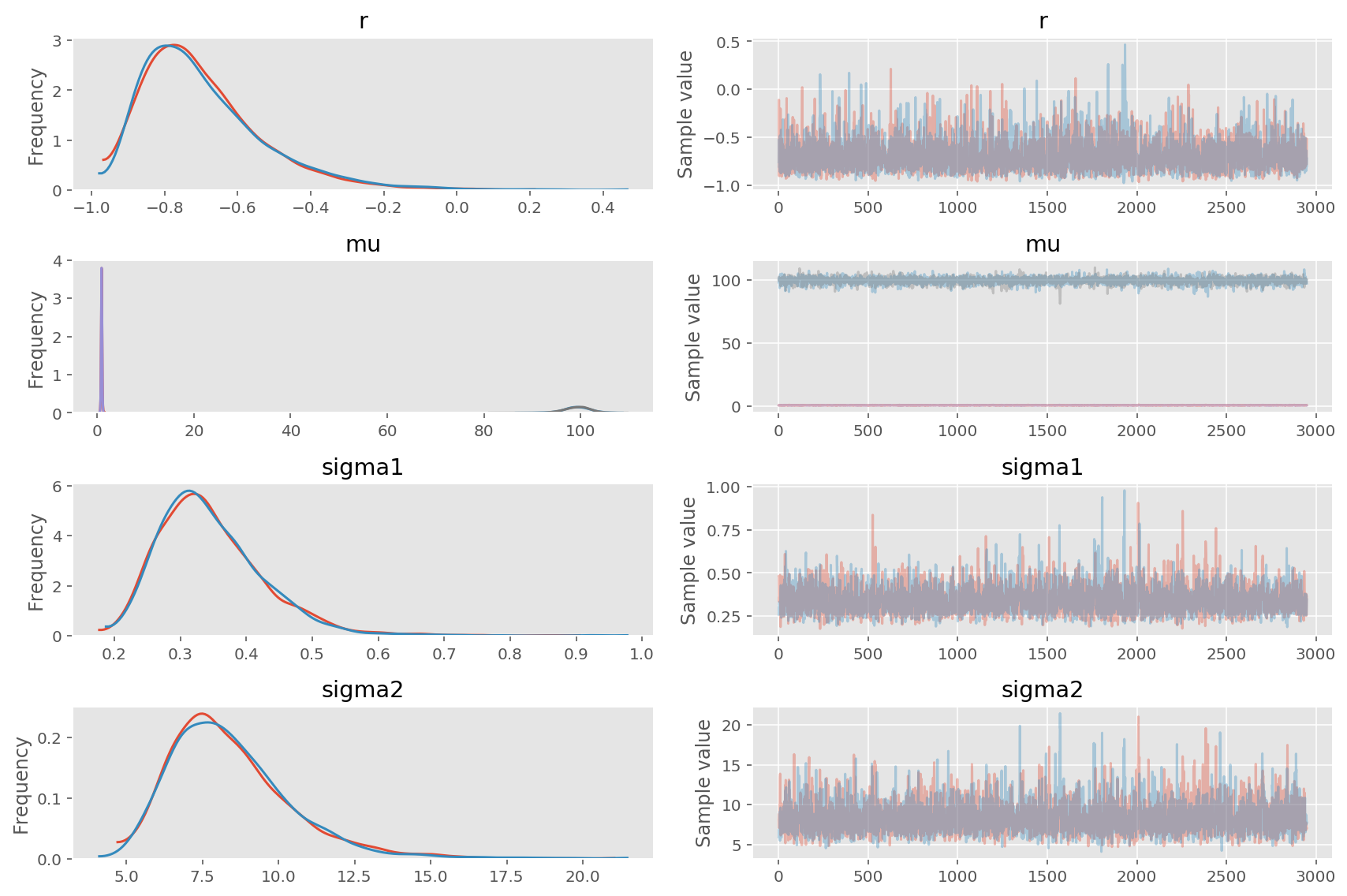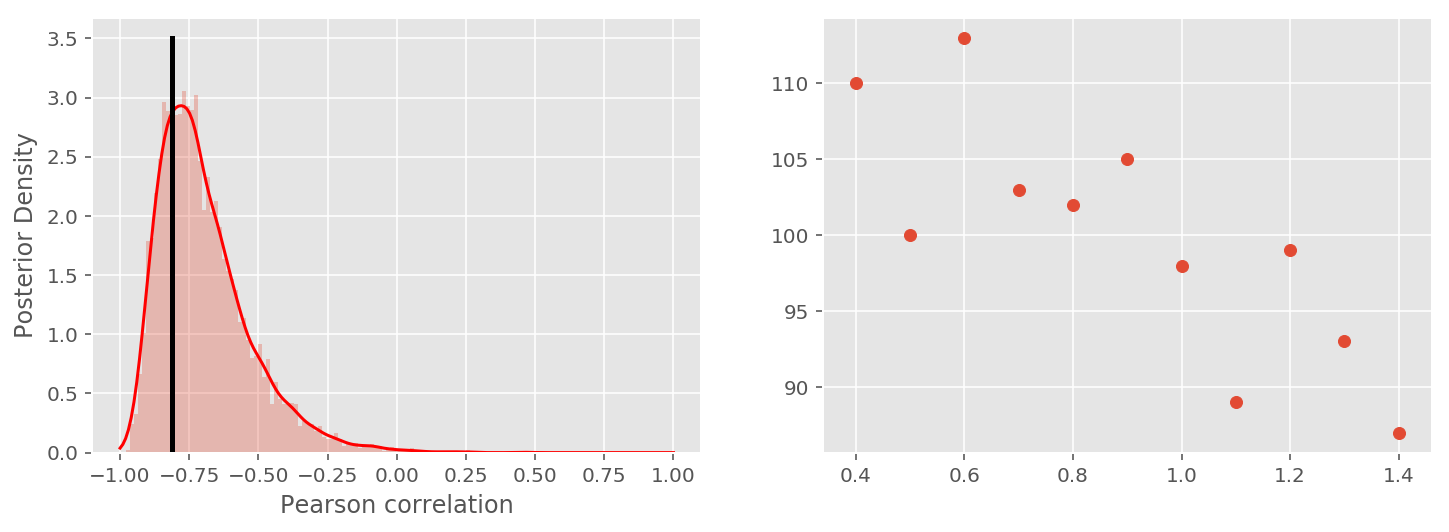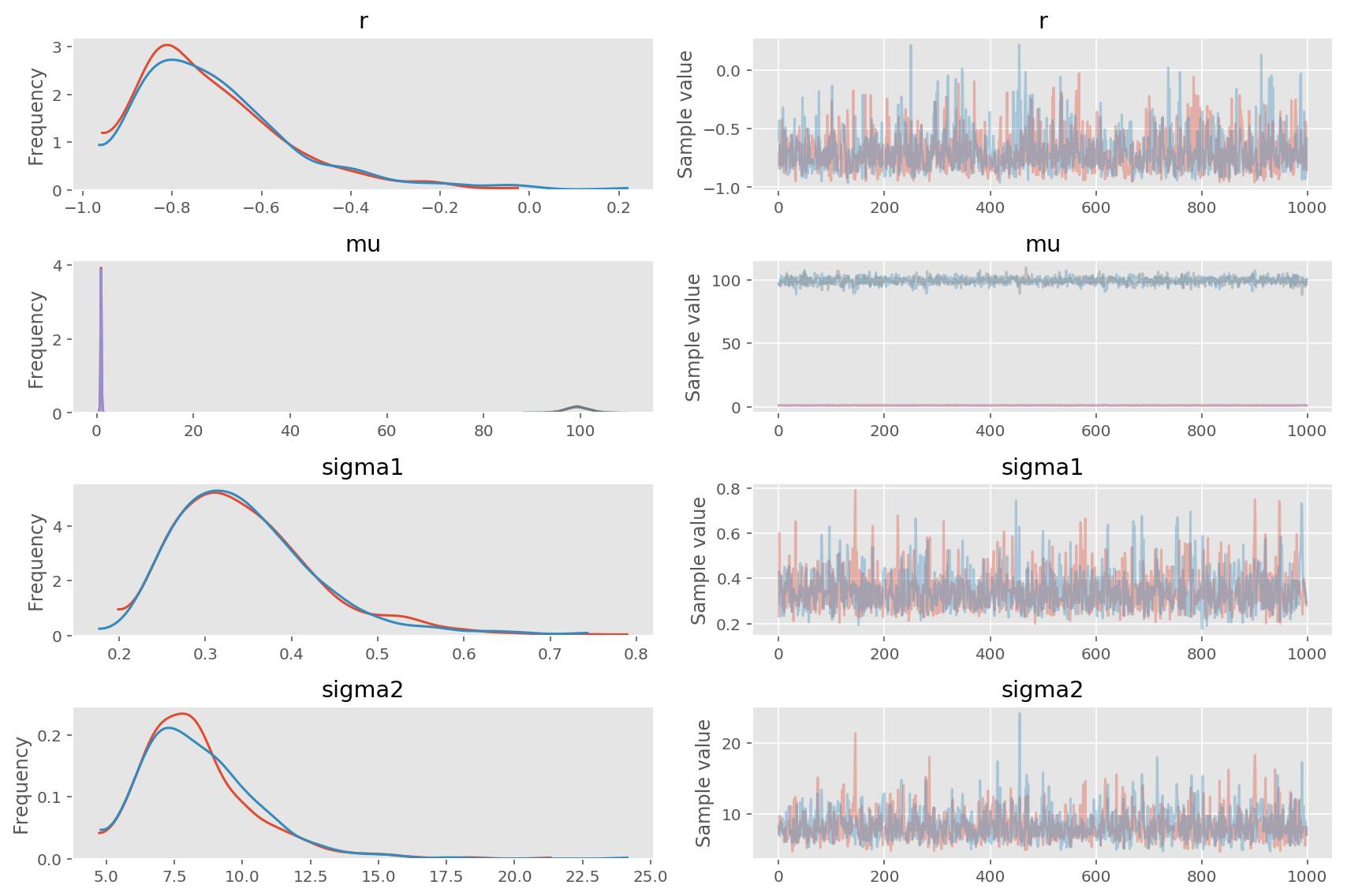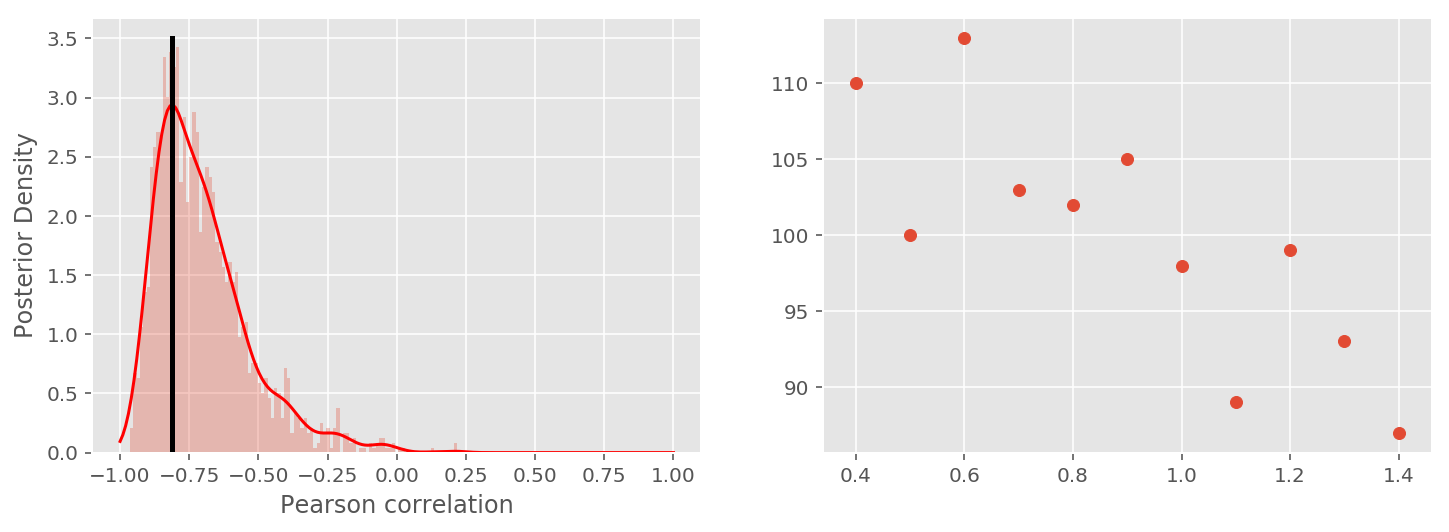In :
import pymc3 as pm
import numpy as np
import pandas as pd
import matplotlib.pyplot as plt

%matplotlib inline
%config InlineBackend.figure_format = 'retina'
%qtconsole --colors=linux
plt.style.use('ggplot')

from matplotlib import gridspec
from theano import tensor as tt
from scipy import stats


# Chapter 5 - Some examples of data analysis¶

## 5.1 Pearson correlation¶

The Pearson product-moment correlation coefficient, usually denoted r, is a widely used measure of the relationship between two variables. It ranges from −1, indicating a perfect negative linear relationship, to +1, indicating a perfect positive relationship. A value of 0 indicates that there is no linear relationship. Usually the correlation r is reported as a single point estimate, perhaps together with a frequentist significance test.

But, rather than just having a single number to measure the correlation, it would be nice to have a posterior distribution for r, saying how likely each possible level of correlation was. There are frequentist confidence interval methods that try to do this, as well as various analytic Bayesian results based on asymptotic approximations (e.g., Donner & Wells, 1986).

Inferring the mean and variance of a Gaussian distribution. $$\mu_{1},\mu_{2} \sim \text{Gaussian}(0, .001)$$ $$\sigma_{1},\sigma_{2} \sim \text{InvSqrtGamma} (.001, .001)$$ $$r \sim \text{Uniform} (-1, 1)$$
$$x_{i} \sim \text{MvGaussian} ((\mu_{1},\mu_{2}), \begin{bmatrix}\sigma_{1}^2 & r\sigma_{1}\sigma_{2}\\r\sigma_{1}\sigma_{2} & \sigma_{2}^2\end{bmatrix}^{-1})$$

The observed data take the form xi = (xi1, xi2) for the ith observation, and, following the theory behind the correlation coefficient, are modeled as draws from a multivariate Gaussian distribution. The parameters of this distribution are the means μ = (μ1,μ2) and standard deviations σ = (σ1,σ2) of the two variables, and the correlation coefficient r that links them.

In :
# The datasets:
y = np.array([.8, 102, 1,98, .5,100, 0.9,105, .7,103,
0.4,110, 1.2,99, 1.4,87, 0.6,113, 1.1,89, 1.3,93]).reshape((11, 2))

# y = np.array([.8,102, 1,98, .5,100, 0.9,105, .7,103,
#                0.4,110, 1.2,99, 1.4,87, 0.6,113, 1.1,89, 1.3,93,
#                .8,102, 1,98, .5,100, 0.9,105, .7,103,
#                0.4,110, 1.2,99, 1.4,87, 0.6,113, 1.1,89, 1.3,93]).reshape((22, 2))

with pm.Model() as model:
# r∼Uniform(−1,1)
r =  pm.Uniform('r', lower=-1, upper=1)

# μ1,μ2∼Gaussian(0,.001)
mu = pm.Normal('mu', mu=0, tau=.001, shape=2)

# σ1,σ2∼InvSqrtGamma(.001,.001)
lambda1 = pm.Gamma('lambda1', alpha=.001, beta=.001)
lambda2 = pm.Gamma('lambda2', alpha=.001, beta=.001)
sigma1 = pm.Deterministic('sigma1', 1/np.sqrt(lambda1))
sigma2 = pm.Deterministic('sigma2', 1/np.sqrt(lambda2))

cov = pm.Deterministic('cov', tt.stacklists([[lambda1**-1, r*sigma1*sigma2],
[r*sigma1*sigma2, lambda2**-1]]))

# xi∼MvGaussian((μ1,μ2),[σ1^2,rσ1σ2;rσ1σ2,σ2^2]^−1)
yd = pm.MvNormal('yd', mu=mu, cov=cov, observed=y,shape=2)

trace2=pm.sample(3e3, njobs=2)

pm.traceplot(trace2[50:], varnames=['r', 'mu', 'sigma1', 'sigma2']);

Auto-assigning NUTS sampler...
100%|██████████| 3500/3500.0 [00:37<00:00, 93.48it/s]In :
from matplotlib import gridspec
from scipy.stats.kde import gaussian_kde
import scipy as scipy

r = trace2['r'][50:]
freqr = scipy.corrcoef(y[:, 0], y[:, 1])
print('The r estimation is: ', np.mean(r, axis=0))
print('The Pearson correlation r is: ', freqr[0, 1])

fig = plt.figure(figsize=(12, 4))
gs = gridspec.GridSpec(1, 2, width_ratios=[1, 1])
ax0 = plt.subplot(gs)
my_pdf = gaussian_kde(r)
x=np.linspace(-1, 1, 200)
ax0.plot(x, my_pdf(x), 'r') # distribution function
ax0.hist(r, bins=100, normed=1, alpha=.3)
ax0.plot([freqr[0, 1], freqr[0, 1]], [0, 3.5], 'k', lw=2.5)
plt.xlabel('Pearson correlation')
plt.ylabel('Posterior Density')

ax1 = plt.subplot(gs)
ax1.scatter(y[:, 0], y[:, 1]);

The r estimation is:  -0.695576221086
The Pearson correlation r is:  -0.810967075636## 5.2 Pearson correlation with uncertainty¶

$$\mu_{1},\mu_{2} \sim \text{Gaussian}(0, .001)$$$$\sigma_{1},\sigma_{2} \sim \text{InvSqrtGamma} (.001, .001)$$$$r \sim \text{Uniform} (-1, 1)$$

$$y_{i} \sim \text{MvGaussian} ((\mu_{1},\mu_{2}), \begin{bmatrix}\sigma_{1}^2 & r\sigma_{1}\sigma_{2}\\r\sigma_{1}\sigma_{2} & \sigma_{2}^2\end{bmatrix}^{-1})$$ $$x_{ij} \sim \text{Gaussian}(y_{ij},\lambda_{j}^e)$$

In :
# The datasets:
y = np.array([.8, 102, 1,98, .5,100, 0.9,105, .7,103,
0.4,110, 1.2,99, 1.4,87, 0.6,113, 1.1,89, 1.3,93]).reshape((11, 2))
# y = np.array([.8,102, 1,98, .5,100, 0.9,105, .7,103,
#                0.4,110, 1.2,99, 1.4,87, 0.6,113, 1.1,89, 1.3,93,
#                .8,102, 1,98, .5,100, 0.9,105, .7,103,
#                0.4,110, 1.2,99, 1.4,87, 0.6,113, 1.1,89, 1.3,93]).reshape((22, 2))

n1, n2 = np.shape(y)
sigmaerror = np.array([.03, 1])
se = np.tile(sigmaerror, (n1, 1))

with pm.Model() as model2:
# r∼Uniform(−1,1)
r = pm.Uniform('r', lower=-1, upper=1)

# μ1,μ2∼Gaussian(0,.001)
mu = pm.Normal('mu', mu=0, tau=.001, shape=n2)

# σ1,σ2∼InvSqrtGamma(.001,.001)
lambda1 = pm.Gamma('lambda1', alpha=.001, beta=.001)
lambda2 = pm.Gamma('lambda2', alpha=.001, beta=.001)
sigma1 = pm.Deterministic('sigma1', 1/np.sqrt(lambda1))
sigma2 = pm.Deterministic('sigma2', 1/np.sqrt(lambda2))

cov = pm.Deterministic('cov', tt.stacklists([[lambda1**-1, r*sigma1*sigma2],
[r*sigma1*sigma2, lambda2**-1]]))

# xi∼MvGaussian((μ1,μ2),[σ1^2,rσ1σ2;rσ1σ2,σ2^2]^−1)
yd = pm.MvNormal('yd', mu=mu, cov=cov, shape=(n1, n2))

xi = pm.Normal('xi', mu=yd , sd=sigmaerror, observed=y)

trace2=pm.sample(1e3, njobs=2)

Auto-assigning NUTS sampler...
100%|██████████| 1500/1500.0 [00:32<00:00, 46.12it/s]

In :
pm.traceplot(trace2, varnames=['r', 'mu', 'sigma1', 'sigma2']);

r = trace2['r']
freqr=scipy.corrcoef(y[:, 0], y[:, 1])
print('The r estimation is: ', np.mean(r, axis=0))
print('The Pearson correlation r is: ', freqr[0, 1])

fig = plt.figure(figsize=(12, 4))
gs = gridspec.GridSpec(1, 2, width_ratios=[1, 1])
ax0 = plt.subplot(gs)
my_pdf = gaussian_kde(r)
x=np.linspace(-1, 1, 200)
ax0.plot(x, my_pdf(x), 'r') # distribution function
ax0.hist(r, bins=100, normed=1, alpha=.3)
ax0.plot([freqr[0, 1], freqr[0, 1]], [0, 3.5], 'k', lw=2.5)
plt.xlabel('Pearson correlation')
plt.ylabel('Posterior Density')

ax1 = plt.subplot(gs)
ax1.scatter(y[:, 0], y[:, 1]);

The r estimation is:  -0.70135557984
The Pearson correlation r is:  -0.810967075636## 5.3 The kappa coefficient of agreement¶

A measurement to decide how well one decision-making method agrees with another. For this problem, when both decision-making methods make n independent assessments, the data y take the form of four counts: a observations where both methods decide “one,” b observations where the objective method decides “one” but the surrogate method decides “zero,” c observations where the objective method decides “zero” but the surrogate method decides “one,” and d observations where both methods decide “zero,” with n = a + b + c + d.

$$\kappa = (\xi-\psi)/(1-\psi)$$$$\xi = \alpha\beta + (1-\alpha) \gamma$$$$\psi = (\pi_{a}+\pi_{b})(\pi_{a}+\pi_{c})+(\pi_{b}+\pi_{d})(\pi_{c}+\pi_{d})$$$$\alpha,\beta,\gamma \sim \text{Beta} (1, 1)$$

$$\pi_{a} = \alpha\beta$$ $$\pi_{b} = \alpha(1-\beta)$$
$$\pi_{c} = (1-\alpha)(1-\gamma)$$
$$\pi_{d} = (1-\alpha)\gamma$$
$$y \sim \text{Multinomial} ([\pi_{a},\pi_{b},\pi_{c},\pi_{d}],n)$$

In :
# CHOOSE a data set:
# Influenza
y = np.array([14, 4, 5, 210])
# Hearing Loss
# y = np.array([20, 7, 103, 417])
# Rare Disease
# y = np.array([0, 0, 13, 157])

with pm.Model() as model1:
# prior
alpha = pm.Beta('alpha', alpha=1, beta=1)
beta = pm.Beta('beta', alpha=1, beta=1)
gamma = pm.Beta('gamma', alpha=1, beta=1)

pi1 = alpha*beta
pi2 = alpha*(1-beta)
pi3 = (1-alpha)*(1-gamma)
pi4 = (1-alpha)*gamma

# Derived Measures
# Rate Surrogate Method Agrees With the Objective Method
xi = alpha*beta+(1-alpha)*gamma

yd = pm.Multinomial('yd', n=y.sum(), p=[pi1, pi2, pi3, pi4], observed=y)

# Rate of Chance Agreement
psi = pm.Deterministic('psi', (pi1+pi2)*(pi1+pi3)+(pi2+pi4)*(pi3+pi4))

# Chance-Corrected Agreement
kappa = pm.Deterministic('kappa', (xi-psi)/(1-psi))

trace=pm.sample(1e3, njobs=2)

pm.traceplot(trace, varnames=['kappa', 'psi']);

# Compare to Cohen's point estimate
n = y.sum()
p0 = (y+y)/n
pe = (((y+y) * (y+y)) + ((y+y) * (y+y))) / n**2
kappa_Cohen = (p0-pe) / (1-pe)

/Library/Frameworks/Python.framework/Versions/3.5/lib/python3.5/site-packages/theano/tensor/basic.py:2105: UserWarning: theano.tensor.round() changed its default from half_away_from_zero to half_to_even to have the same default as NumPy. Use the Theano flag warn.round=False to disable this warning.
"theano.tensor.round() changed its default from"
Auto-assigning NUTS sampler...